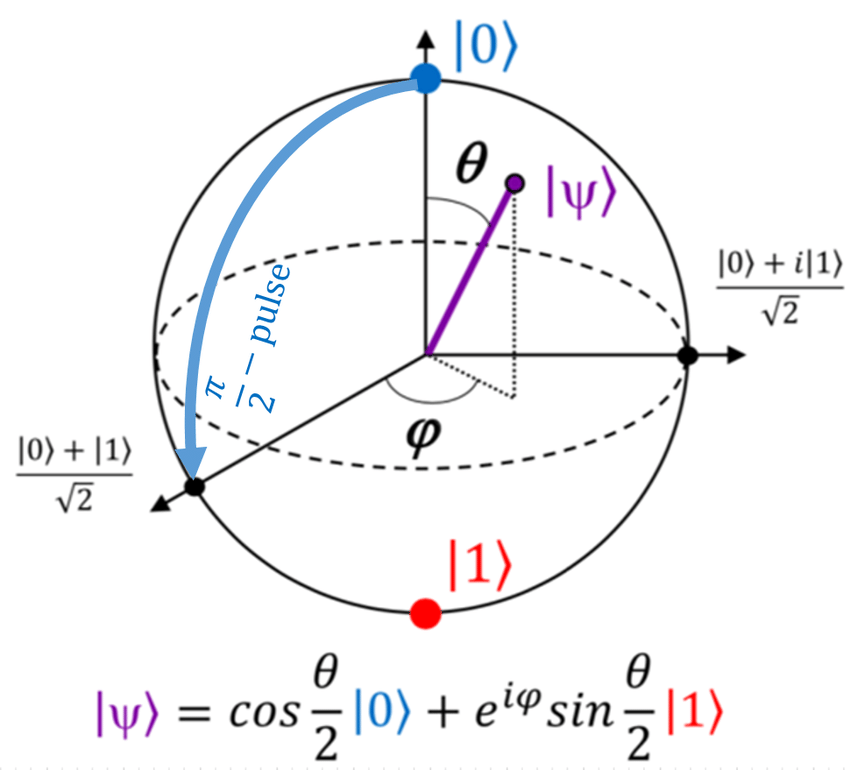# 一些量子计算的东西

## 量子

### 波函数 (Wave function)

#### 波函数叠加 (Superposition)

##### 叠加态

$|\psi\rangle=c_{1}|a_1\rangle+c_{2}|a_2\rangle$

## 量子计算

### 数学

#### 布洛赫球面 (Bloch sphere)$|\psi\rangle=a_{0}|0\rangle+a_{1}|1\rangle$

$P( |0\rangle ) = |a_0|^2$
$P( |1\rangle ) = |a_1|^2$

#### 来点线代

$$|0\rangle = \begin{bmatrix} 1 \ 0 \end{bmatrix}$$

$$|1\rangle = \begin{bmatrix} 0 \ 1 \end{bmatrix}$$

$$|\psi\rangle = a_0 \begin{bmatrix} 1 \ 0 \end{bmatrix} + a_1 \begin{bmatrix} 0 \ 1 \end{bmatrix} = \begin{bmatrix} a_0 \ a_1 \end{bmatrix}$$

#### 多比特测量

$|\psi\rangle=a|00\rangle+b|01\rangle+c|10\rangle+d|11\rangle$

$|\psi\rangle \otimes |\psi^{\prime}\rangle = |\psi\rangle|\psi^{\prime}\rangle = |\psi\psi^{\prime}\rangle$

$\begin{bmatrix} a_0 \ a_1 \end{bmatrix} \otimes \begin{bmatrix} b_0 \ b_1 \end{bmatrix} = \begin{bmatrix} a_0b_0 \ a_0b_1 \ a_1b_0 \ a_1b_1 \end{bmatrix}$

$|00\rangle = \begin{bmatrix} 1 \ 0 \ 0 \ 0 \end{bmatrix}$

$|01\rangle = \begin{bmatrix} 0 \ 1 \ 0 \ 0 \end{bmatrix}$

$|10\rangle = \begin{bmatrix} 0 \ 0 \ 1 \ 0 \end{bmatrix}$

$|11\rangle = \begin{bmatrix} 0 \ 0 \ 0 \ 1 \end{bmatrix}$

$|\phi\rangle^{+} = \frac{1}{\sqrt{2}}(|00\rangle + |11\rangle)$